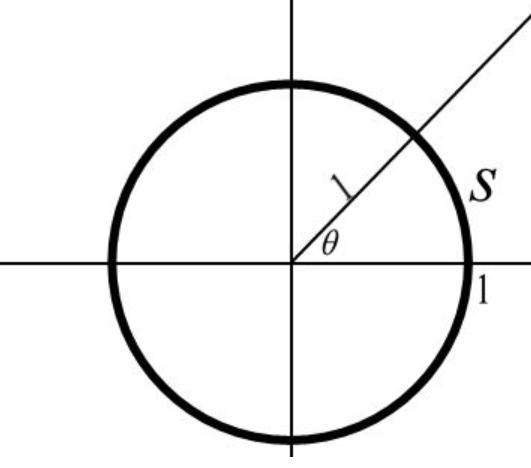×
Get Full Access to Calculus: Early Transcendentals - 1 Edition - Chapter 1.4 - Problem 3e
Get Full Access to Calculus: Early Transcendentals - 1 Edition - Chapter 1.4 - Problem 3e

×

# Ch 1.4 - 3EISBN: 9780321570567 2

## Solution for problem 3E Chapter 1.4

Calculus: Early Transcendentals | 1st Edition

• Textbook Solutions
• 2901 Step-by-step solutions solved by professors and subject experts
• Get 24/7 help from StudySoup virtual teaching assistantsCalculus: Early Transcendentals | 1st Edition

4 5 1 331 Reviews
19
2
Problem 3E

Explain how the radian measure of an angle is determined?

Step-by-Step Solution:

Step 1 of 3

Consider the circle  of radius 1 and length of arc,We need to find how the radian measure of an angle is determined.

Step 2 of 3

Step 3 of 3

##### ISBN: 9780321570567

The full step-by-step solution to problem: 3E from chapter: 1.4 was answered by , our top Calculus solution expert on 03/03/17, 03:45PM. Since the solution to 3E from 1.4 chapter was answered, more than 362 students have viewed the full step-by-step answer. The answer to “?Explain how the radian measure of an angle is determined?” is broken down into a number of easy to follow steps, and 10 words. This textbook survival guide was created for the textbook: Calculus: Early Transcendentals, edition: 1. This full solution covers the following key subjects: . This expansive textbook survival guide covers 85 chapters, and 5218 solutions. Calculus: Early Transcendentals was written by and is associated to the ISBN: 9780321570567.

Unlock Textbook Solution